# ArviZ.jl Quickstart

Note

This tutorial is adapted from ArviZ's quickstart.

## Setup

Here we add the necessary packages for this notebook and load a few we will use throughout.

using ArviZ, CmdStan, Distributions, LinearAlgebra, PyPlot, Random, Turing
# ArviZ ships with style sheets!
ArviZ.use_style("arviz-darkgrid")

## Get started with plotting

ArviZ.jl is designed to be used with libraries like CmdStan, Turing.jl, and Soss.jl but works fine with raw arrays.

rng1 = Random.MersenneTwister(37772);
begin
plot_posterior(randn(rng1, 100_000))
gcf()
end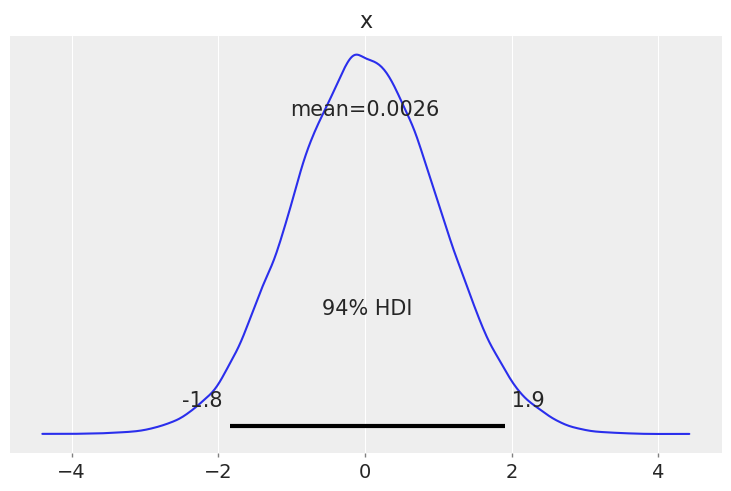Plotting a dictionary of arrays, ArviZ.jl will interpret each key as the name of a different random variable. Each row of an array is treated as an independent series of draws from the variable, called a chain. Below, we have 10 chains of 50 draws each for four different distributions.

let
s = (10, 50)
plot_forest((
normal=randn(rng1, s),
gumbel=rand(rng1, Gumbel(), s),
student_t=rand(rng1, TDist(6), s),
exponential=rand(rng1, Exponential(), s),
),)
gcf()
end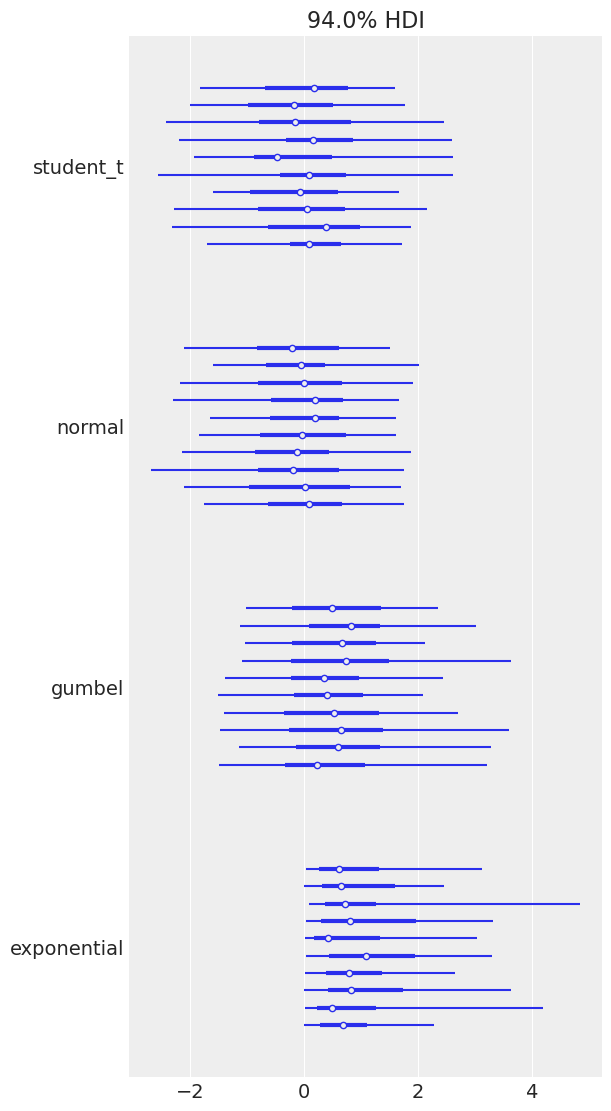## Plotting with MCMCChains.jl's Chains objects produced by Turing.jl

ArviZ is designed to work well with high dimensional, labelled data. Consider the eight schools model, which roughly tries to measure the effectiveness of SAT classes at eight different schools. To show off ArviZ's labelling, I give the schools the names of a different eight schools.

This model is small enough to write down, is hierarchical, and uses labelling. Additionally, a centered parameterization causes divergences (which are interesting for illustration).

First we create our data and set some sampling parameters.

begin
J = 8
y = [28.0, 8.0, -3.0, 7.0, -1.0, 1.0, 18.0, 12.0]
σ = [15.0, 10.0, 16.0, 11.0, 9.0, 11.0, 10.0, 18.0]
schools = [
"Choate",
"Deerfield",
"Phillips Andover",
"Phillips Exeter",
"Hotchkiss",
"Lawrenceville",
"St. Paul's",
"Mt. Hermon",
]
ndraws = 1_000
ndraws_warmup = 1_000
nchains = 4
end;

Now we write and run the model using Turing:

Turing.@model function model_turing(y, σ, J=length(y))
μ ~ Normal(0, 5)
τ ~ truncated(Cauchy(0, 5), 0, Inf)
θ ~ filldist(Normal(μ, τ), J)
for i in 1:J
y[i] ~ Normal(θ[i], σ[i])
end
end
model_turing (generic function with 3 methods)
rng2 = Random.MersenneTwister(16653);
begin
param_mod_turing = model_turing(y, σ)
sampler = NUTS(ndraws_warmup, 0.8)

turing_chns = Turing.sample(
rng2, model_turing(y, σ), sampler, MCMCThreads(), ndraws, nchains
)
end;

Most ArviZ functions work fine with Chains objects from Turing:

begin
plot_autocorr(turing_chns; var_names=(:μ, :τ))
gcf()
end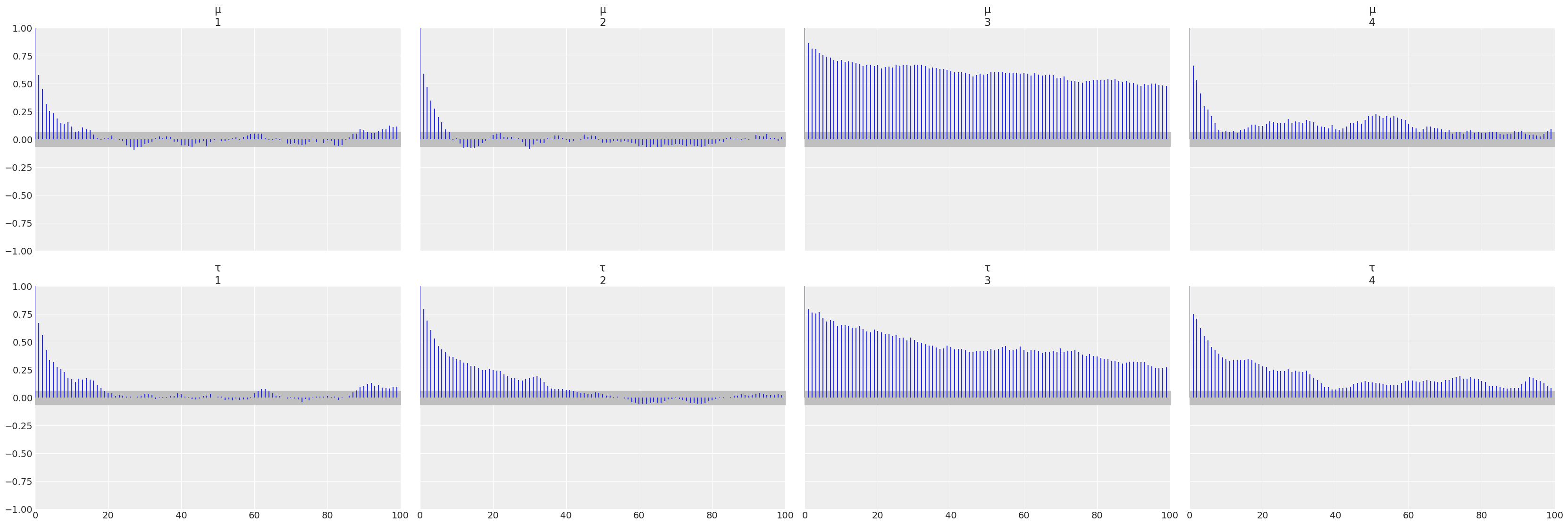### Convert to InferenceData

For much more powerful querying, analysis and plotting, we can use built-in ArviZ utilities to convert Chains objects to multidimensional data structures with named dimensions and indices. Note that for such dimensions, the information is not contained in Chains, so we need to provide it.

ArviZ is built to work with InferenceData, and the more groups it has access to, the more powerful analyses it can perform.

idata_turing_post = from_mcmcchains(
turing_chns;
coords=(; school=schools),
dims=NamedTuple(k => (:school,) for k in (:y, :σ, :θ)),
library="Turing",
)
InferenceData
posterior
Dataset with dimensions:
Dim{:chain} Sampled Base.OneTo(4) ForwardOrdered Regular Points,
Dim{:draw} Sampled Base.OneTo(1000) ForwardOrdered Regular Points,
Dim{:school} Categorical String[Choate, Deerfield, …, St. Paul's, Mt. Hermon] Unordered
and 3 layers:
:μ Float64 dims: Dim{:chain}, Dim{:draw} (4×1000)
:τ Float64 dims: Dim{:chain}, Dim{:draw} (4×1000)
:θ Float64 dims: Dim{:chain}, Dim{:draw}, Dim{:school} (4×1000×8)

with metadata OrderedCollections.OrderedDict{Symbol, Any} with 2 entries:
:created_at => "2022-09-02T21:22:44.877"
:inference_library => "Turing"
sample_stats
Dataset with dimensions:
Dim{:chain} Sampled Base.OneTo(4) ForwardOrdered Regular Points,
Dim{:draw} Sampled Base.OneTo(1000) ForwardOrdered Regular Points
and 12 layers:
:energy           Float64 dims: Dim{:chain}, Dim{:draw} (4×1000)
:n_steps          Int64 dims: Dim{:chain}, Dim{:draw} (4×1000)
:diverging        Bool dims: Dim{:chain}, Dim{:draw} (4×1000)
:max_energy_error Float64 dims: Dim{:chain}, Dim{:draw} (4×1000)
:energy_error     Float64 dims: Dim{:chain}, Dim{:draw} (4×1000)
:is_accept        Bool dims: Dim{:chain}, Dim{:draw} (4×1000)
:log_density      Float64 dims: Dim{:chain}, Dim{:draw} (4×1000)
:tree_depth       Int64 dims: Dim{:chain}, Dim{:draw} (4×1000)
:step_size        Float64 dims: Dim{:chain}, Dim{:draw} (4×1000)
:acceptance_rate  Float64 dims: Dim{:chain}, Dim{:draw} (4×1000)
:lp               Float64 dims: Dim{:chain}, Dim{:draw} (4×1000)
:step_size_nom    Float64 dims: Dim{:chain}, Dim{:draw} (4×1000)

with metadata OrderedCollections.OrderedDict{Symbol, Any} with 2 entries:
:created_at => "2022-09-02T21:22:44.619"
:inference_library => "Turing"

Each group is an ArviZ.Dataset, a DimensionalData.AbstractDimStack that can be used identically to a DimensionalData.Dimstack. We can view a summary of the dataset.

idata_turing_post.posterior
Dataset with dimensions:
Dim{:chain} Sampled Base.OneTo(4) ForwardOrdered Regular Points,
Dim{:draw} Sampled Base.OneTo(1000) ForwardOrdered Regular Points,
Dim{:school} Categorical String[Choate, Deerfield, …, St. Paul's, Mt. Hermon] Unordered
and 3 layers:
:μ Float64 dims: Dim{:chain}, Dim{:draw} (4×1000)
:τ Float64 dims: Dim{:chain}, Dim{:draw} (4×1000)
:θ Float64 dims: Dim{:chain}, Dim{:draw}, Dim{:school} (4×1000×8)

with metadata OrderedCollections.OrderedDict{Symbol, Any} with 2 entries:
:created_at        => "2022-09-02T21:22:44.877"
:inference_library => "Turing"

Here is a plot of the trace. Note the intelligent labels.

begin
plot_trace(idata_turing_post)
gcf()
end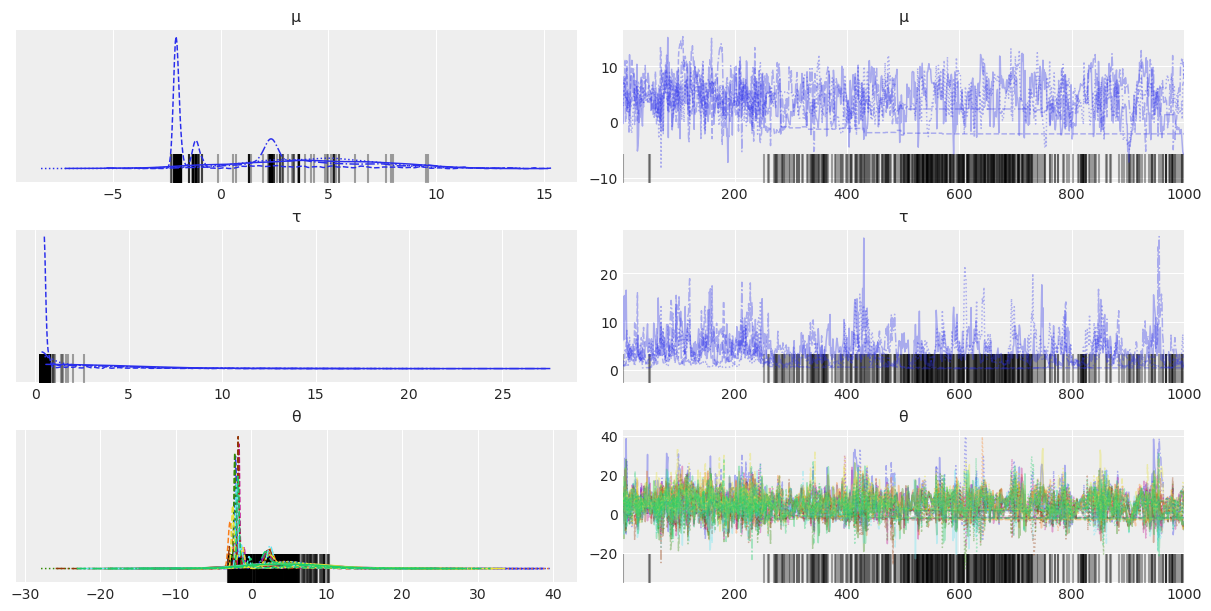We can also generate summary stats...

summarystats(idata_turing_post)
variable mean sd hdi_3% hdi_97% mcse_mean mcse_sd ess_bulk ess_tail r_hat
1 "μ" 3.063 3.796 -2.332 9.667 1.054 0.763 13.0 6.0 1.22
2 "τ" 3.037 3.13 0.3 8.565 0.618 0.442 11.0 11.0 1.29
3 "θ[Choate]" 4.575 6.173 -3.159 16.998 1.396 1.002 14.0 7.0 1.2
4 "θ[Deerfield]" 3.441 5.05 -3.274 13.345 1.236 0.89 15.0 6.0 1.19
5 "θ[Phillips Andover]" 2.615 5.248 -5.27 13.417 0.914 0.652 28.0 815.0 1.1
6 "θ[Phillips Exeter]" 3.385 4.934 -3.745 13.297 1.055 0.756 20.0 1198.0 1.13
7 "θ[Hotchkiss]" 2.423 4.621 -4.002 12.224 0.856 0.611 27.0 1124.0 1.1
8 "θ[Lawrenceville]" 2.73 4.917 -4.622 12.842 0.921 0.658 23.0 952.0 1.11
9 "θ[St. Paul's]" 4.728 5.845 -2.462 16.305 1.477 1.065 12.0 6.0 1.23
10 "θ[Mt. Hermon]" 3.426 5.541 -4.58 15.213 1.065 0.761 21.0 1177.0 1.12

...and examine the energy distribution of the Hamiltonian sampler.

begin
plot_energy(idata_turing_post)
gcf()
end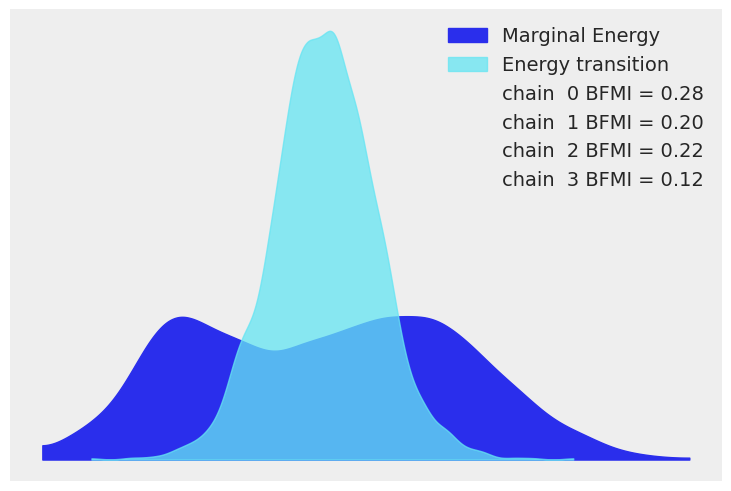### Additional information in Turing.jl

With a few more steps, we can use Turing to compute additional useful groups to add to the InferenceData.

To sample from the prior, one simply calls sample but with the Prior sampler:

prior = Turing.sample(rng2, param_mod_turing, Prior(), ndraws);

To draw from the prior and posterior predictive distributions we can instantiate a "predictive model", i.e. a Turing model but with the observations set to missing, and then calling predict on the predictive model and the previously drawn samples:

begin
# Instantiate the predictive model
param_mod_predict = model_turing(similar(y, Missing), σ)
# and then sample!
prior_predictive = Turing.predict(rng2, param_mod_predict, prior)
posterior_predictive = Turing.predict(rng2, param_mod_predict, turing_chns)
end;

And to extract the pointwise log-likelihoods, which is useful if you want to compute metrics such as loo,

log_likelihood = let
log_likelihood = Turing.pointwise_loglikelihoods(
param_mod_turing, MCMCChains.get_sections(turing_chns, :parameters)
)
# Ensure the ordering of the loglikelihoods matches the ordering of posterior_predictive
ynames = string.(keys(posterior_predictive))
log_likelihood_y = getindex.(Ref(log_likelihood), ynames)
# Reshape into (nchains, ndraws, size(y)...)
(; y=permutedims(cat(log_likelihood_y...; dims=3), (2, 1, 3)))
end;

This can then be included in the from_mcmcchains call from above:

idata_turing = from_mcmcchains(
turing_chns;
posterior_predictive,
log_likelihood,
prior,
prior_predictive,
observed_data=(; y),
coords=(; school=schools),
dims=NamedTuple(k => (:school,) for k in (:y, :σ, :θ)),
library=Turing,
)
InferenceData
posterior
Dataset with dimensions:
Dim{:chain} Sampled Base.OneTo(4) ForwardOrdered Regular Points,
Dim{:draw} Sampled Base.OneTo(1000) ForwardOrdered Regular Points,
Dim{:school} Categorical String[Choate, Deerfield, …, St. Paul's, Mt. Hermon] Unordered
and 3 layers:
:μ Float64 dims: Dim{:chain}, Dim{:draw} (4×1000)
:τ Float64 dims: Dim{:chain}, Dim{:draw} (4×1000)
:θ Float64 dims: Dim{:chain}, Dim{:draw}, Dim{:school} (4×1000×8)

with metadata OrderedCollections.OrderedDict{Symbol, Any} with 3 entries:
:created_at => "2022-09-02T21:23:37.124"
:inference_library => "Turing"
:inference_library_version => "0.21.10"
posterior_predictive
Dataset with dimensions:
Dim{:chain} Sampled Base.OneTo(4) ForwardOrdered Regular Points,
Dim{:draw} Sampled Base.OneTo(1000) ForwardOrdered Regular Points,
Dim{:school} Categorical String[Choate, Deerfield, …, St. Paul's, Mt. Hermon] Unordered
and 1 layer:
:y Float64 dims: Dim{:chain}, Dim{:draw}, Dim{:school} (4×1000×8)

with metadata OrderedCollections.OrderedDict{Symbol, Any} with 3 entries:
:created_at => "2022-09-02T21:23:36.491"
:inference_library => "Turing"
:inference_library_version => "0.21.10"
log_likelihood
Dataset with dimensions:
Dim{:chain} Sampled Base.OneTo(4) ForwardOrdered Regular Points,
Dim{:draw} Sampled Base.OneTo(1000) ForwardOrdered Regular Points,
Dim{:school} Categorical String[Choate, Deerfield, …, St. Paul's, Mt. Hermon] Unordered
and 1 layer:
:y Float64 dims: Dim{:chain}, Dim{:draw}, Dim{:school} (4×1000×8)

with metadata OrderedCollections.OrderedDict{Symbol, Any} with 3 entries:
:created_at => "2022-09-02T21:23:36.849"
:inference_library => "Turing"
:inference_library_version => "0.21.10"
sample_stats
Dataset with dimensions:
Dim{:chain} Sampled Base.OneTo(4) ForwardOrdered Regular Points,
Dim{:draw} Sampled Base.OneTo(1000) ForwardOrdered Regular Points
and 12 layers:
:energy           Float64 dims: Dim{:chain}, Dim{:draw} (4×1000)
:n_steps          Int64 dims: Dim{:chain}, Dim{:draw} (4×1000)
:diverging        Bool dims: Dim{:chain}, Dim{:draw} (4×1000)
:max_energy_error Float64 dims: Dim{:chain}, Dim{:draw} (4×1000)
:energy_error     Float64 dims: Dim{:chain}, Dim{:draw} (4×1000)
:is_accept        Bool dims: Dim{:chain}, Dim{:draw} (4×1000)
:log_density      Float64 dims: Dim{:chain}, Dim{:draw} (4×1000)
:tree_depth       Int64 dims: Dim{:chain}, Dim{:draw} (4×1000)
:step_size        Float64 dims: Dim{:chain}, Dim{:draw} (4×1000)
:acceptance_rate  Float64 dims: Dim{:chain}, Dim{:draw} (4×1000)
:lp               Float64 dims: Dim{:chain}, Dim{:draw} (4×1000)
:step_size_nom    Float64 dims: Dim{:chain}, Dim{:draw} (4×1000)

with metadata OrderedCollections.OrderedDict{Symbol, Any} with 3 entries:
:created_at => "2022-09-02T21:23:37.124"
:inference_library => "Turing"
:inference_library_version => "0.21.10"
prior
Dataset with dimensions:
Dim{:chain} Sampled Base.OneTo(1) ForwardOrdered Regular Points,
Dim{:draw} Sampled Base.OneTo(1000) ForwardOrdered Regular Points,
Dim{:school} Categorical String[Choate, Deerfield, …, St. Paul's, Mt. Hermon] Unordered
and 3 layers:
:μ Float64 dims: Dim{:chain}, Dim{:draw} (1×1000)
:τ Float64 dims: Dim{:chain}, Dim{:draw} (1×1000)
:θ Float64 dims: Dim{:chain}, Dim{:draw}, Dim{:school} (1×1000×8)

with metadata OrderedCollections.OrderedDict{Symbol, Any} with 3 entries:
:created_at => "2022-09-02T21:23:38.521"
:inference_library => "Turing"
:inference_library_version => "0.21.10"
prior_predictive
Dataset with dimensions:
Dim{:chain} Sampled Base.OneTo(1) ForwardOrdered Regular Points,
Dim{:draw} Sampled Base.OneTo(1000) ForwardOrdered Regular Points,
Dim{:school} Categorical String[Choate, Deerfield, …, St. Paul's, Mt. Hermon] Unordered
and 1 layer:
:y Float64 dims: Dim{:chain}, Dim{:draw}, Dim{:school} (1×1000×8)

with metadata OrderedCollections.OrderedDict{Symbol, Any} with 3 entries:
:created_at => "2022-09-02T21:23:37.926"
:inference_library => "Turing"
:inference_library_version => "0.21.10"
sample_stats_prior
Dataset with dimensions:
Dim{:chain} Sampled Base.OneTo(1) ForwardOrdered Regular Points,
Dim{:draw} Sampled Base.OneTo(1000) ForwardOrdered Regular Points
and 1 layer:
:lp Float64 dims: Dim{:chain}, Dim{:draw} (1×1000)

with metadata OrderedCollections.OrderedDict{Symbol, Any} with 3 entries:
:created_at => "2022-09-02T21:23:38.234"
:inference_library => "Turing"
:inference_library_version => "0.21.10"
observed_data
Dataset with dimensions:
Dim{:school} Categorical String[Choate, Deerfield, …, St. Paul's, Mt. Hermon] Unordered
and 1 layer:
:y Float64 dims: Dim{:school} (8)

with metadata OrderedCollections.OrderedDict{Symbol, Any} with 3 entries:
:created_at => "2022-09-02T21:23:39.113"
:inference_library => "Turing"
:inference_library_version => "0.21.10"

Then we can for example compute the expected leave-one-out (LOO) predictive density, which is an estimate of the out-of-distribution predictive fit of the model:

loo(idata_turing; pointwise=false) # higher is better
loo loo_se p_loo n_samples n_data_points warning loo_scale
1 -31.2192 1.60441 1.0423 4000 8 true "log"

If the model is well-calibrated, i.e. it replicates the true generative process well, the CDF of the pointwise LOO values should be similarly distributed to a uniform distribution. This can be inspected visually:

begin
plot_loo_pit(idata_turing; y=:y, ecdf=true)
gcf()
end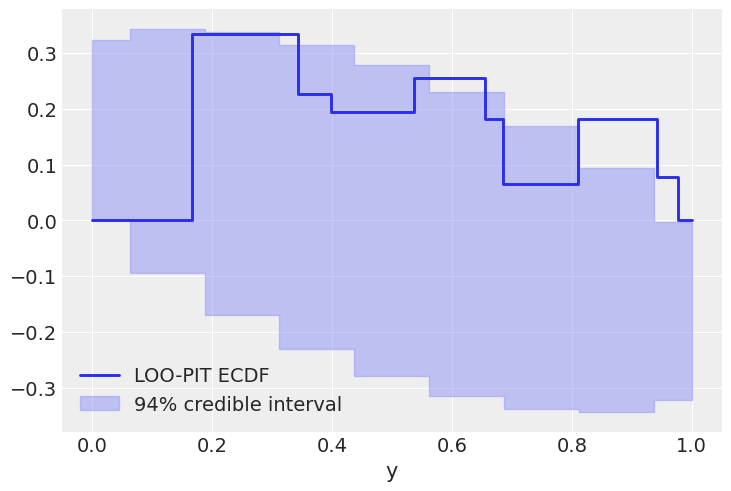## Plotting with CmdStan.jl outputs

CmdStan.jl and StanSample.jl also default to producing Chains outputs, and we can easily plot these chains.

Here is the same centered eight schools model:

begin
schools_code = """
data {
int<lower=0> J;
real y[J];
real<lower=0> sigma[J];
}

parameters {
real mu;
real<lower=0> tau;
real theta[J];
}

model {
mu ~ normal(0, 5);
tau ~ cauchy(0, 5);
theta ~ normal(mu, tau);
y ~ normal(theta, sigma);
}

generated quantities {
vector[J] log_lik;
vector[J] y_hat;
for (j in 1:J) {
log_lik[j] = normal_lpdf(y[j] | theta[j], sigma[j]);
y_hat[j] = normal_rng(theta[j], sigma[j]);
}
}
"""

schools_data = Dict("J" => J, "y" => y, "sigma" => σ)
stan_chns = mktempdir() do path
stan_model = Stanmodel(;
model=schools_code,
name="schools",
nchains,
num_warmup=ndraws_warmup,
num_samples=ndraws,
output_format=:mcmcchains,
random=CmdStan.Random(28983),
tmpdir=path,
)
_, chns, _ = stan(stan_model, schools_data; summary=false)
return chns
end
end;
begin
plot_density(stan_chns; var_names=(:mu, :tau))
gcf()
end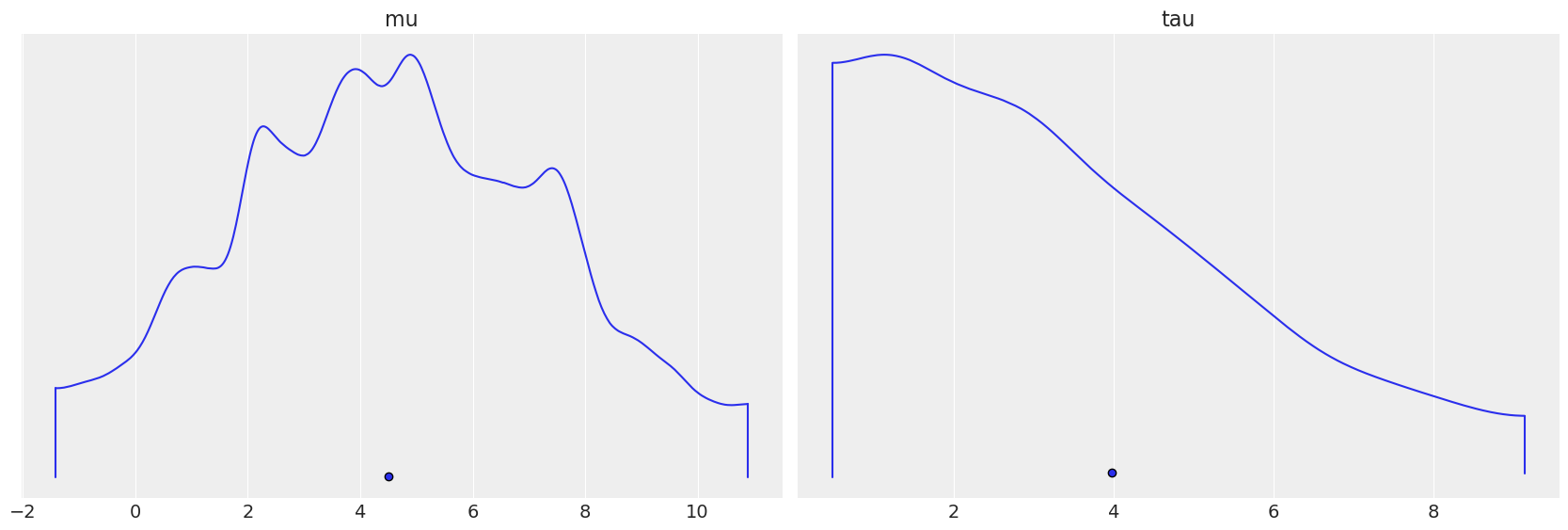Again, converting to InferenceData, we can get much richer labelling and mixing of data. Note that we're using the same from_cmdstan function used by ArviZ to process cmdstan output files, but through the power of dispatch in Julia, if we pass a Chains object, it instead uses ArviZ.jl's overloads, which forward to from_mcmcchains.

idata_stan = from_cmdstan(
stan_chns;
posterior_predictive=:y_hat,
observed_data=(; y),
log_likelihood=:log_lik,
coords=(; school=schools),
dims=NamedTuple(k => (:school,) for k in (:y, :sigma, :theta, :log_lik, :y_hat)),
)
InferenceData
posterior
Dataset with dimensions:
Dim{:chain} Sampled Base.OneTo(4) ForwardOrdered Regular Points,
Dim{:draw} Sampled Base.OneTo(1000) ForwardOrdered Regular Points,
Dim{:school} Categorical String[Choate, Deerfield, …, St. Paul's, Mt. Hermon] Unordered
and 3 layers:
:mu    Float64 dims: Dim{:chain}, Dim{:draw} (4×1000)
:theta Float64 dims: Dim{:chain}, Dim{:draw}, Dim{:school} (4×1000×8)
:tau   Float64 dims: Dim{:chain}, Dim{:draw} (4×1000)

with metadata OrderedCollections.OrderedDict{Symbol, Any} with 2 entries:
:created_at => "2022-09-02T21:24:32.671"
:inference_library => "CmdStan"
posterior_predictive
Dataset with dimensions:
Dim{:chain} Sampled Base.OneTo(4) ForwardOrdered Regular Points,
Dim{:draw} Sampled Base.OneTo(1000) ForwardOrdered Regular Points,
Dim{:school} Categorical String[Choate, Deerfield, …, St. Paul's, Mt. Hermon] Unordered
and 1 layer:
:y_hat Float64 dims: Dim{:chain}, Dim{:draw}, Dim{:school} (4×1000×8)

with metadata OrderedCollections.OrderedDict{Symbol, Any} with 2 entries:
:created_at => "2022-09-02T21:24:31.703"
:inference_library => "CmdStan"
log_likelihood
Dataset with dimensions:
Dim{:chain} Sampled Base.OneTo(4) ForwardOrdered Regular Points,
Dim{:draw} Sampled Base.OneTo(1000) ForwardOrdered Regular Points,
Dim{:school} Categorical String[Choate, Deerfield, …, St. Paul's, Mt. Hermon] Unordered
and 1 layer:
:log_lik Float64 dims: Dim{:chain}, Dim{:draw}, Dim{:school} (4×1000×8)

with metadata OrderedCollections.OrderedDict{Symbol, Any} with 2 entries:
:created_at => "2022-09-02T21:24:32.108"
:inference_library => "CmdStan"
sample_stats
Dataset with dimensions:
Dim{:chain} Sampled Base.OneTo(4) ForwardOrdered Regular Points,
Dim{:draw} Sampled Base.OneTo(1000) ForwardOrdered Regular Points
and 7 layers:
:tree_depth      Int64 dims: Dim{:chain}, Dim{:draw} (4×1000)
:n_steps         Int64 dims: Dim{:chain}, Dim{:draw} (4×1000)
:lp              Float64 dims: Dim{:chain}, Dim{:draw} (4×1000)
:step_size       Float64 dims: Dim{:chain}, Dim{:draw} (4×1000)
:energy          Float64 dims: Dim{:chain}, Dim{:draw} (4×1000)
:diverging       Bool dims: Dim{:chain}, Dim{:draw} (4×1000)
:acceptance_rate Float64 dims: Dim{:chain}, Dim{:draw} (4×1000)

with metadata OrderedCollections.OrderedDict{Symbol, Any} with 2 entries:
:created_at => "2022-09-02T21:24:32.671"
:inference_library => "CmdStan"
observed_data
Dataset with dimensions:
Dim{:school} Categorical String[Choate, Deerfield, …, St. Paul's, Mt. Hermon] Unordered
and 1 layer:
:y Float64 dims: Dim{:school} (8)

with metadata OrderedCollections.OrderedDict{Symbol, Any} with 2 entries:
:created_at => "2022-09-02T21:24:33.195"
:inference_library => "CmdStan"

Here is a plot showing where the Hamiltonian sampler had divergences:

begin
plot_pair(
idata_stan;
coords=Dict(:school => ["Choate", "Deerfield", "Phillips Andover"]),
divergences=true,
)
gcf()
end